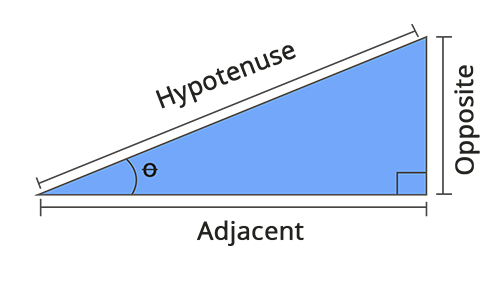• Call Now

1800-102-2727•

# Trigonometry Table: Formulas and Functions

Trigonometry was first introduced by the Greek mathematician named Hipparchus. Trigonometry and its functions are primarily classified into three main functions. They are sine, cosine, and tangent. This topic will discuss the relation between the sides and angles of a right-angled triangle and basic trigonometric formulae, functions, and ratios, etc.

Trigonometry is a mathematical function that helps us find the missing angles and sides of a triangle with the help of ratios called trigonometric ratios. The angles can easily be measured in degrees. The following are the commonly used trigonometric angles: 0°, 30°, 45°, 60°, and 90° (check the trigonometry chart for more details). There are two sub-branches in trigonometry. They are plane trigonometry and spherical geometry.

Trigonometric functions are nothing but the trigonometric ratios of a triangle. Sine, cosine and tangent are the three basic trigonometric functions. The abbreviations of these functions are sin, cos and tan. The following is an example of these functions in a right-angled triangle. The longest side of a right-angled triangle is called the hypotenuse side. Likewise, the sides opposite the hypotenuse side are termed the opposite side and the adjacent side.Given below are the formulae for 6 important trigonometric functions:
sin θ = opposite side / hypotenuse side
cos θ = adjacent side / hypotenuse side
tan θ = opposite side / adjacent side
cosec θ =  hypotenuse side / opposite side
sec θ = hypotenuse side / adjacent side
cot θ = adjacent side / opposite side

Trigonometric ratios can be calculated using the given 6 formulae. Therefore, it is a fundamental need to get enough knowledge regarding the sides of the right-angled triangle because it describes important trigonometric functions.

Apart from all this, a new concept called the unit circle is introduced to help us measure the angles of sin, cos and tan directly. This is because the circle's centre is located at the origin, and the radius is 1. Thus, if the perpendicular distance is y, and the base is x, the length of the hypotenuse can be equivalent to the radius of the unit circle, i.e. 1.Hence, the trigonometric ratios can be expressed as follows,
Sin = y/1 = y
Cos = x/1 = x
Tan = y/x

Trigonometry can be used broadly and has many real-time examples. The following is a basic example, If the height of some building is given and the angle formed when an object is observed from the building's top, then the distance between the object and the bottom of the building can be demonstrated with the help of a tangent function, namely the tan of an angle is equal to the ratio of the building height and the distance. Let us consider the angle , then,
Tan = height / distance between the object and the building Distance = height /tan α
The following are some of the applications of trigonometry,

• Very helpful in various fields such as seismology, navigation, astronomy, oceanography, meteorology, acoustics, scientific research etc.
• It is useful while measuring the mountain height, finding the length of long rivers etc.Talk to our expert
Resend OTP Timer =
By submitting up, I agree to receive all the Whatsapp communication on my registered number and Aakash terms and conditions and privacy policy